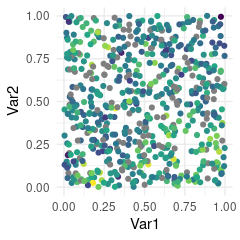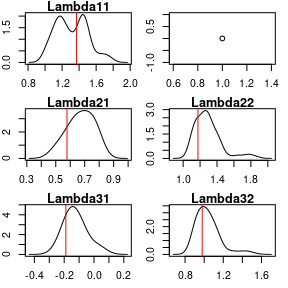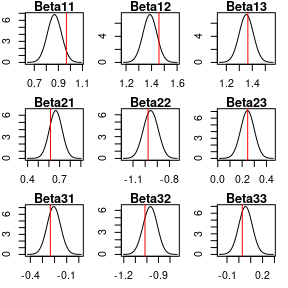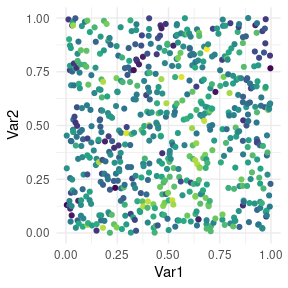# MGPs for multivariate data at irregularly spaced locations

#### 2022-09-19

This is very similar to the univariate, irregularly spaced data case. The only difference is in formatting the data and in having to specify how many spatial factors should be used. NOTE: there are strict limits to how much time this vignette can take up on CRAN’s servers as they are a shared resource. For a somewhat better example, consider setting SS = 30 to generate the data, then run the MCMC chain for much longer, use block_size=20 or larger, increase n_threads according to available resources.

library(magrittr)
library(dplyr)
library(ggplot2)
library(meshed)

set.seed(2021)

SS <- 25 # coord values for jth dimension
dd <- 2 # spatial dimension
n <- SS^2 # number of locations
q <- 3 # number of outcomes
k <- 2 # true number of spatial factors used to make the outcomes
p <- 3 # number of covariates

coords <- cbind(runif(n), runif(n)) %>%
as.data.frame()
colnames(coords) <- c("Var1", "Var2")

clist <- 1:q %>% lapply(function(i) coords %>%
mutate(mv_id=i) %>%
as.matrix())

philist <- c(5, 10)

# cholesky decomp of covariance matrix
LClist <- 1:k %>% lapply(function(i) t(chol(
exp(-philist[i] * as.matrix(dist(clist[[i]])) ))))

# generating the factors
wlist <- 1:k %>% lapply(function(i) LClist[[i]] %*% rnorm(n))

# factor matrix
WW <- do.call(cbind, wlist)

Lambda <- matrix(0, q, ncol(WW))
diag(Lambda) <- runif(k, 1, 2)
Lambda[lower.tri(Lambda)] <- runif(sum(lower.tri(Lambda)), -1, 1)

# nuggets
tau.sq <- rep(.05, q)
TTsq <- matrix(1, nrow=n) %x% matrix(tau.sq, ncol=length(tau.sq))
# measurement errors
EE <- ( rnorm(n*length(tau.sq)) %>% matrix(ncol=length(tau.sq)) ) * TTsq^.5

XX <- matrix(rnorm(n*p), ncol=p)
Beta <- matrix(rnorm(p*q), ncol=q)

# outcome matrix, fully observed
YY_full <- XX %*% Beta + WW %*% t(Lambda) + EE

# .. introduce some NA values in the outcomes
# all at different locations
YY <- YY_full
for(i in 1:q){
YY[sample(1:n, n/5, replace=FALSE), i] <- NA
}

Let’s plot the second outcome for example.

simdata <- coords %>%
cbind(data.frame(Outcome_full=YY_full,
Outcome_obs = YY))

simdata %>%
ggplot(aes(Var1, Var2, color=Outcome_obs.2)) +
geom_point() + scale_color_viridis_c() +
theme_minimal() + theme(legend.position="none")We target the estimation of the following regression model, for data at spatial location $$s$$: $Y(s) = X(s)\beta + \Lambda v(s) + E(s)$ where $$Y(s)$$ is of dimension $$q$$, $$X(s)$$ has $$q$$ rows and $$pq$$ columns, $$\beta$$ has dimension $$pq$$ (we can represent it as matrix with $$q$$ rows and $$p$$ columns), $$\Lambda$$ is a matrix with $$q$$ rows and $$k$$ columns, $$v(s)$$ is a $$k$$ dimensional vector of spatial random effects, and $$E(s)$$ has dimension $$q$$ and is assumed to be Gaussian with diagonal covariance matrix. If we sample $$v(s)$$ at a grid of knots, the model above becomes $Y(s) = X(s)\beta + \Lambda H v(s) + E'(s),$ where we adjust via $$H$$ and the variance of $$E'(s)$$. The second model is fit by using settings$forced_grid=TRUE as in the univariate case. The spmeshed function works the same way as before, but we need to specify $$k$$ unless we seek to fit the model with $$k=q$$. We store the outcomes in YY, one in each column. These can be NA when missing. spmeshed works as in the univariate case, but we can now choose how many factors to use (default is k=ncol(y)) mcmc_keep <- 200 # too small! this is just a vignette. mcmc_burn <- 400 mcmc_thin <- 2 mesh_total_time <- system.time({ meshout <- spmeshed(y=YY, x=XX, coords=coords, k = 2, grid_size = c(20, 20), block_size = 16, n_samples = mcmc_keep, n_burn = mcmc_burn, n_thin = mcmc_thin, n_threads = 2, prior = list(phi=c(2, 20)), verbose=0 )}) Some post-processing. Let’s look at the factor loadings $$\Lambda$$. True values in red. plot_cube(meshout$lambda_mcmc, ncol(YY), 2, Lambda, "Lambda")Regression coefficients for each outcome.

plot_cube(meshout$beta_mcmc, ncol(YY), p, Beta, "Beta", T)And finally some map of predictions using posterior means. mcmc_summary <- function(x) c(quantile(x, .025), mean(x), quantile(x, .975)) y_post_sample <- meshout$yhat_mcmc %>%
abind::abind(along=3) %>%
apply(1:2, mcmc_summary)

# posterior mean for 3rd outcome:
meshout$coordsdata %>% cbind(y_pm_3 = y_post_sample[2,,3]) %>% filter(forced_grid==0) %>% ggplot(aes(Var1, Var2, color=y_pm_3)) + geom_point() + scale_color_viridis_c() + theme_minimal() + theme(legend.position="none")Let’s compute the correlation between our predictions for the third outcome at its unobserved locations, and the true values which we generated in the simulated dataset. perf <- meshout$coordsdata %>%
cbind(y_pm_3 = y_post_sample[2,,3]) %>%
left_join(simdata, by = c("Var1", "Var2"))

perf %>% filter(forced_grid==0, !complete.cases(Outcome_obs.3)) %>%
with(cor(y_pm_3, Outcome_full.3))
#>  0.9645428